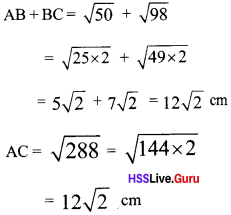# Kerala Syllabus 9th Standard Maths Solutions Chapter 4 New Numbers

## Kerala State Syllabus 9th Standard Maths Solutions Chapter 4 New Numbers

### Kerala Syllabus 9th Standard Maths New Numbers Text Book Questions and Answers

Textbook Page No. 49

New Numbers Class 9 Kerala Syllabus Chapter 4 Question 1.
In the picture, the square on the hypotenuse of the top most right triangle is drawn. Calculate the area and the length of a side of the square.Hypotenuse of first right triangle
$$\sqrt { { 1 }^{ 2 }+{ 1 }^{ 2 } } =\sqrt { {1} + {1} } = \sqrt {2}$$
Hypotenuse of second right triangle
$$\sqrt {\sqrt {{2}}^{ 2 } + 1^{2}} = \sqrt {2 + 1} = \sqrt {3}$$
Hypotenuse of third right triangle
$$\sqrt {\sqrt { { 3 } }^{ 2 } + 1^{ 2 }} = \sqrt {3 + 1} =\sqrt {4}$$ = 2
Hypotenuse of fourth right triangle
$$\sqrt { { 2 }^{ 2 }+{ 1 }^{ 2 } } =\sqrt { { 4 } + { 1 } } =\sqrt { 5 }$$
i. e. the length of one side of square is $$\sqrt 5$$
Area $$\sqrt 5$$ × $$\sqrt 5$$ = 5 sq. m

Class 9 Maths Chapter 4 Kerala Syllabus Question 2.
A square is drawn on the altitude of an equilateral triangle of side 2m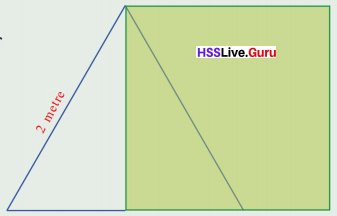(i) What is the area of the square?
(ii) What is the altitude of the triangle?
iii) What are the lengths of the other two sides of the triangle shown below?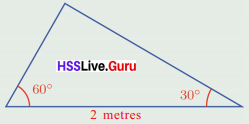(i)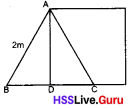BD = 1 m
AD = $$\sqrt { { 2 }^{ 2 }-{ 1 }^{ 2 } } = \sqrt { { 4 }-{ 1 } } = \sqrt { 3 }$$
Area of square
= $$\sqrt 3$$ × $$\sqrt 3$$ = 3 sq. m

(ii) Height of triangle $$\sqrt 3$$cm

(iii)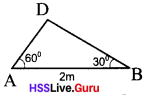Sides are 1 m and $$\sqrt 3$$ m
Sides opposite to 30° angle = 1 m
Sides opposite to 60° = $$\sqrt 3$$ m

Kerala Syllabus 9th Standard Maths Chapter 4 Question 3.
We have seen in Class 8 that any odd number can be written as the difference of two perfect squares. (The lesson, Identities). Using this, draw squares of areas 7 and 11 square centimetres.
3² – 2² = 5
4² – 3² = 7
5² – 4² = 9
n² – (n – 1)² = 2n – 1
so 4² – 3² = 7
6² – 5² = 11
Draw a right angled triangle with hypotenuse 4cm and one of its side as 3 cm. It’s one side is $$\sqrt 7$$ cmDraw square BCDE with BC as the side.Area of square BCDE = $$\sqrt 7$$ × $$\sqrt 7$$ = 7 sq.cm
Draw a right angled triangle with hypotenuse 6 cm and one of its side as 5 cm. It’s one side is $$\sqrt 11$$ cm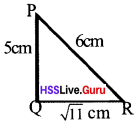Draw square BCDE with BC as the side.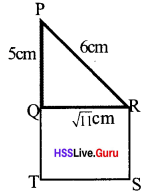Area of square QRST = $$\sqrt 11$$ × $$\sqrt 11$$ = 11 sq.m

Class 9 Maths Chapter 4 New Numbers Kerala Syllabus Question 4.
Explain two different methods of drawing a square of area 13 square centimetres.
Method 1
72 – 62 = 13 Draw a right angled triangle with one side 6 cm and hypotenuse 7 cm.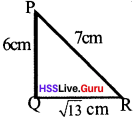Draw square QRST with QR as the side.Method 2.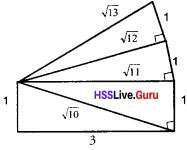Draw a right angled triangle with 1, 3 as perpendicular sides. Hypotenuse is $$\sqrt 10$$
Draw a right angled triangle with $$\sqrt 10$$, 1, as perpendicular sides. Hypotenuse is $$\sqrt 11$$
Draw a right angled triangle with $$\sqrt 11$$, 1 as perpendicular sides. Hypotenuse is $$\sqrt 12$$
Draw a right angled triangle with $$\sqrt 12$$, 1 as perpendicular sides. Hypotenuse is $$\sqrt 13$$. If AB = $$\sqrt 13$$ cm.
Draw a square ABCD with AB as side.9th Standard Maths Chapter 4 Kerala Syllabus Question 5.
Find three fractions larger than $$\sqrt 2$$ and less than $$\sqrt 3$$
$$\sqrt 2$$ = 1.41; $$\sqrt 3$$ = 1.73
Numbers in between $$\sqrt 2$$ and $$\sqrt 3$$ are 1.5, 1.6, 1.65 So fractions are $$\frac {15}{10}$$, $$\frac {16}{10}$$, $$\frac {165}{100}$$

Textbook Page No. 52

Kerala Syllabus 9th Standard Maths Notes Chapter 4 Question 1.
The hypotenuse of a right triangle is 1$$\frac {1}{2}$$ m and another side is $$\frac {1}{2}$$ m. Calculate its perimeter correct to a centimetre.
Square of the third side is =
$$(1\frac {1}{2})^{2} – (\frac {1}{2})^{2} = (1\frac {1}{2} + \frac {1}{2}) (1\frac {1}{2} – \frac {1}{2})$$ = 2 × 1 = 2
∴ Third side is $$\sqrt 2$$
Perimeter = 1$$\frac {1}{2}$$ + $$\frac {1}{2}$$ + $$\sqrt 2$$ = 2 + $$\sqrt 2$$
= 2 + 1.41 = 3.412 m = 341.2 cm

New Numbers Class 9 Questions And Answers Kerala Syllabus Chapter 4 Question 2.
The picture shows an equilateral triangle cut into halves by a line through a vertex.
i. What is the perimeter of a part? (See the second problem at the end of the previous section)
ii. How much less than the perimeter of the whole triangle is this?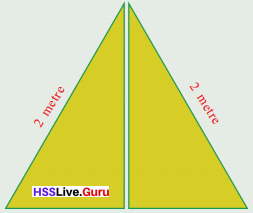The sides of the new triangle is 2 cm,
1 m, $$\sqrt {2}^{2}-{1}^{2}$$ m
(i) Perimeter of one of the triangle =
2 + 1 +$$\sqrt 3$$ = 3 + 1.73 = 4.73 m

(ii) Perimeter of the whole triangle
= 2 + 2 + 2 = 6m
Less in the perimeter
= 6 – 4.73 = 1.27m

9th Class Maths Notes Chapter 4 Kerala Syllabus Chapter 4 Question 3.
Calculate the perimeter of the triangle shown below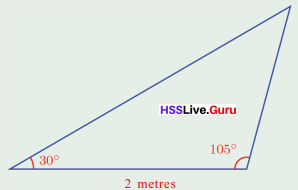Draw BD perpendicular to ACAB = 2;
BD= 1;
AD= $$\sqrt 3$$
BD = 1, DC = 1:
BC = $$\sqrt 2$$
AC $$\sqrt 3$$ + 1; AB = 2: BC = $$\sqrt 2$$
Perimeter = $$\sqrt 3$$ + 1 + 2 + $$\sqrt 2$$
= 3 + $$\sqrt 3$$ + $$\sqrt 2$$
= 3 + 1.73 + 1.41 = 6.14 m

Hsslive Guru 9th Maths Kerala Syllabus Chapter 4 Question 4.
We have seen how we can draw a series of right triangles as in the picture.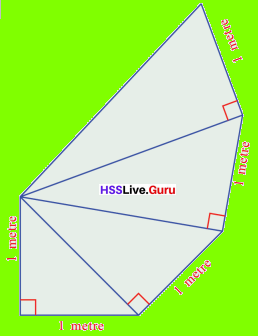(i) What are the lengths of the sides of the tenth triangle drawn like this?
(ii) How much more is the perimeter of the tenth triangle than the perimeter of the ninth triangle?
(iii) How do we write in algebra, the difference in perimeter of the n‘h triangle and that of the triangle just before it?
i. Sides of the first triangle = 1 m, 1 m, $$\sqrt 2$$ m
Sides of the second triangle = 1 m, $$\sqrt 2$$ m, $$\sqrt 3$$ m
Sides of the third triangle = 1 m, $$\sqrt 3$$ m, $$\sqrt 4$$ m
…………………………
…………………………
Sides of the tenth triangle = 1 m, $$\sqrt 10$$ m, $$\sqrt 11$$ m
Hypotenuse of the 10th triangle is $$\sqrt 11$$
Perpendicular sides are $$\sqrt 10$$, 1

(ii) Perimeter of tenth triangle
= 1 m + $$\sqrt 10$$ m + $$\sqrt 11$$ m
Perimeter of ninth triangle = 1 m + $$\sqrt 9$$ m + $$\sqrt 10$$ m
More in the perimeter
= ($$\sqrt 10$$ + $$\sqrt 11$$ + 1) – ($$\sqrt 9$$ + $$\sqrt 10$$ + 1)
= $$\sqrt 11$$ – $$\sqrt 9$$ = ($$\sqrt 11$$ – 3)m

iii. Sides of the nth triangle are
$$\sqrt n$$, $$\sqrt n + 1$$, 1
The sides of the (n – 1)th triangle are
$$\sqrt n – 1$$, $$\sqrt n$$, 1
The difference in perimeter
= ($$\sqrt n + 1$$) – ($$\sqrt n – 1$$)

Hsslive Guru Maths 9th Kerala Syllabus Chapter 4 Question 5.
What is the hypotenuse of the right triangle with perpendicular sides $$\sqrt 2$$ centimetres and $$\sqrt 3$$ centimetres? How much larger than the hypotenuse is the sum of the perpendicular sides?
Hypotenuse = $$\sqrt {\sqrt 3^{2}} + {\sqrt 2^{2}}$$
= $$\sqrt 3 + 2$$ = $$\sqrt 5$$ cm
Sum of perpendicular sides = $$\sqrt 3$$ + $$\sqrt 2$$ Difference with the hypotenuse
= ($$\sqrt 3$$ + $$\sqrt 2$$) – $$\sqrt 5$$
= (1.73 + 1.41) – 2.24 = 3.14 – 2.24 cm = 0.9

Textbook Page No. 57

Hsslive Guru Class 9 Maths Kerala Syllabus Chapter 4 Question 1.
Of four equal equilateral triangles, two cut vertically into halves and two whole are put together to make a rectangle.If a side of the triangle is 1 m, what is the area and perimeter of the rectangle?
Sides of the triangle are $$\frac {1}{2}, \frac{\sqrt 3}{2}$$, 1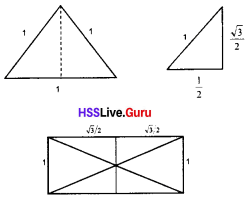Perimeter = 1 + $$\frac{\sqrt 3}{2}$$ + $$\frac{\sqrt 3}{2}$$ + 1 + $$\frac{\sqrt 3}{2}$$ + $$\frac{\sqrt 3}{2}$$
= 2 + 2$$\sqrt 3$$ m
Area of the rectangle = 1 × $$\sqrt 3$$ = $$\sqrt 3$$ m²

Hsslive Class 9 Maths Kerala Syllabus Chapter 4 Question 2.
A square and an equilateral triangle of sides twice as long are cut and the pieces are rearranged to form a trapezium, as shown below:
If a side of the square is 2 cm, what are the perimeter and area of the trapezium?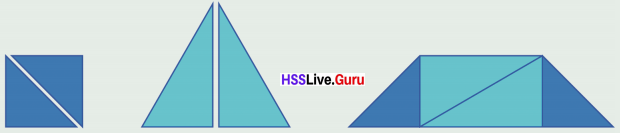Perimeter = 2 + 2$$\sqrt 3$$ + 2 + 2$$\sqrt 2$$ + 2$$\sqrt 3$$ + 2$$\sqrt 2$$
= 4 + 4$$\sqrt 3$$ + 4$$\sqrt 2$$ = 4(1 + $$\sqrt 3$$ + $$\sqrt 2$$)
Area = 2² + $$\frac {1}{2}$$ × 4 × 2$$\sqrt 3$$ = 4 + 4$$\sqrt 3$$
= 4(1 + $$\sqrt 3$$) = 4(1 + 1.73)
= 4 × 2.73 = 10.92 cm

9th Maths Notes Kerala Syllabus Chapter 4 Question 3.
Calculate the perimeter and area of the triangle in the picture.Draw a perpendicular from B to AC
AB = 4cmDB = 2$$\sqrt 3$$
DB = DC = 2$$\sqrt 3$$
BC = $$\sqrt {12} + {12}$$ = $$\sqrt 24$$
Perimeter =
= 4 + $$\sqrt 24$$ + 2$$\sqrt 3$$ + 2
= 6 + $$\sqrt 24$$ + 2$$\sqrt 3$$ cm
Area = $$\frac {1}{2}$$ × AC × DB
= $$\frac {1}{2}$$ × (2 + 2$$\sqrt 3$$) × 2$$\sqrt 3$$ = (2 + 2$$\sqrt 3$$) $$\sqrt 3$$
= 2$$\sqrt 3$$ + 2$$\sqrt 3$$ × $$\sqrt 3$$ = 2$$\sqrt 3$$ + 6
= 6 + 2$$\sqrt 3$$

Kerala Syllabus 9th Standard Maths Notes Pdf Chapter 4 Question 4.
From the pairs of numbers given below, pick out those whose product is a natural number or a fraction.
i. $$\sqrt 3$$, $$\sqrt 12$$
ii. $$\sqrt 3$$, $$\sqrt 1.2$$
iii. $$\sqrt 5$$, $$\sqrt 8$$
iv. $$\sqrt 0.5$$, $$\sqrt 8$$
v. $$\sqrt {7\frac {1}{2}}$$, $$\sqrt 3{\frac{1}{3}}$$
i. $$\sqrt 3$$, $$\sqrt 12$$
$$\sqrt 3$$ × $$\sqrt 12$$ = $$\sqrt 3 × 12$$ = $$\sqrt 36$$ = 6
product is natural number

ii. $$\sqrt 3$$, $$\sqrt 1.2$$
$$\sqrt 3$$ × $$\sqrt 1.2$$ = $$\sqrt 3 × 1.2$$ = $$\sqrt 3.6$$
product is neither a natural number nor a fraction

iii. $$\sqrt 5$$, $$\sqrt 8$$
$$\sqrt 5$$ × $$\sqrt 8$$ = $$\sqrt 5 × 8$$ = $$\sqrt 40$$
product is neither a natural number nor a fraction

iv. $$\sqrt 0.5$$, $$\sqrt 8$$
$$\sqrt 0.5$$ × $$\sqrt 8$$ = $$\sqrt 0.5 × 8$$ = $$\sqrt 4$$ = 2
product is natural number

v. $$\sqrt {7\frac {1}{2}}$$, $$\sqrt 3{\frac{1}{3}}$$
$$\sqrt {7\frac {1}{2}}$$ × $$\sqrt 3{\frac{1}{3}}$$ = $$\sqrt {7\frac {15}{2}}$$ × $$\sqrt 3{\frac{10}{3}}$$
= $$\sqrt {7\frac {150}{6}}$$ = $$\sqrt 25$$ = 5
product is natural number

Textbook Page No. 60

9th Standard Maths Notes Kerala Syllabus Chapter 4 Question 1.
Calculate the length of the sides of the equilateral triangle on the right, correct to a millimetre.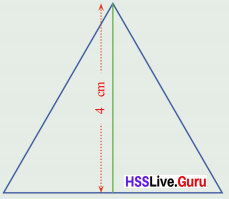Let one side = 2x
$$\sqrt (2x)^{2} – x^{2}$$ = 4
$$\sqrt 4x^{2} – x^{2}$$ = 4;
$$\sqrt 3x^{2}$$ = 4; $$\sqrt 3$$ x = 4; x = $$\frac {4}{\sqrt 3}$$ = 4;
x = $$\frac{4 \sqrt{3}}{\sqrt{3} \times \sqrt{3}} =\frac{4}{3} \sqrt{3} =\frac{4}{3} \times 1.73 = 2.309$$cm
= 2.31 cm
Length of sides = 2 × $$\frac {4}{\sqrt 3}$$ = 2 × 2.309
= 4.62 cm = 46.2 cm

Question 2.
Prove that ($$(\sqrt 2 + 1)(\sqrt 2 – 1 )$$) = 1. Use this to compute $$\frac {1}{\sqrt {2} – 1}$$ correct to two decimal places.
$$(\sqrt{2} + 1)(\sqrt{2} – 1) = (\sqrt{2})^{2} – 1^{2}=2 – 1 = 1$$
$$(\sqrt 2 + 1)(\sqrt 2 – 1 )$$ = 1
$$\frac{1}{\sqrt{2} – 1} = \frac{1}{\sqrt{2} – 1} \times \frac{\sqrt{2} + 1}{\sqrt{2} + 1} = \frac{\sqrt{2} + 1}{1} = \sqrt{2} + 1$$
$$\frac{1}{\sqrt{2} – 1} = \sqrt {2} + 1$$ = 1.41 + 1 = 2.41

Question 3.
Compute $$\frac{1}{\sqrt{2} + 1}$$ corrects to two decimal places.
$$\frac{1}{\sqrt{2} + 1} = \frac{1}{\sqrt{2} + 1} \times \frac{\sqrt{2} – 1}{\sqrt{2} – 1} = \frac{\sqrt{2} – 1}{1}$$
= $$\sqrt {2}$$ – 1 = 1.41 – 1 = 0.41

Question 4.
Simplify ($$\sqrt 3 – \sqrt 2$$)($$\sqrt 3 + \sqrt 2$$). Use this to compute $$\frac{1}{\sqrt{3} – \sqrt {2}}$$ and $$\frac{1}{\sqrt{3} + \sqrt {2}}$$ correct to two decimal places.
(a – b)(a+b) = a² – b²
$$(\sqrt{3} – \sqrt{2})(\sqrt{3} + \sqrt{2}) = (\sqrt{3})^{2} – (\sqrt{2})^{2}$$
= 3 – 2 = 1
$$\frac{1}{\sqrt{3} – \sqrt{2}} = \frac{(\sqrt{3} -\sqrt{2})(\sqrt{3} + \sqrt{2})}{(\sqrt{3} – \sqrt{2})} = \sqrt{3} + \sqrt{2}$$
≈ 1.732 + 1.414 = 3.146 = 3.15
$$\frac{1}{\sqrt{3} + \sqrt{2}} = \frac{(\sqrt{3} – \sqrt{2})(\sqrt{3} + \sqrt{2})}{(\sqrt{3} + \sqrt{2})}=\sqrt{3} – \sqrt{2}$$
≈ 1.732 – 1.414 = 0.318 = 0.32

Question 5.
Prove that $$\sqrt{2 \frac{2}{3}} = 2 \sqrt{\frac{2}{3}}$$. Can you find other numbers like this?
$$\sqrt{2 \frac{2}{3}} = \sqrt{\frac{8}{3}} = \sqrt{4 \times \frac{2}{3}} = 2 \sqrt{\frac{2}{3}}$$
$$\sqrt{3 \frac{3}{8}} = \sqrt{\frac{27}{8}} = \sqrt{9 \times \frac{3}{8}} = 3 \sqrt{\frac{3}{8}}$$
If $$\sqrt{x \frac{x}{n}} = x \sqrt{\frac{x}{n}}$$ then
$$\sqrt{x + \frac{x}{n}} = \sqrt{x^{2} \frac{x}{n}} = \sqrt{\frac{x^{3}}{n}}$$
$$x + \frac{x}{n} = \frac{x^{3}}{n}$$
nx + x = x³
n + 1 = x²
n = x² – 1
similar numbers are
$$4 \frac{4}{4^{2} – 1} = 4 \frac{4}{15}$$
$$5 \frac{5}{5^{2} – 1} = 5 \frac{5}{24}$$
$$\sqrt{4 \frac{4}{15}} = 4 \sqrt{\frac{4}{15}}$$
$$\sqrt{5 \frac{5}{15}} = 5 \sqrt{\frac{5}{15}}$$ etc.

Question 6.
All red triangles in the picture are equilateral. What is the ratio of the sides of the outer and inner squares?Let the sides of the shaded triangles are a units. The sides of the unshaded triangles are a, a, $$4 \frac{a}{2}\sqrt 3$$ units.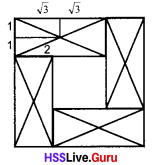Side of the outer square = $$\sqrt{3}\frac{a}{2} + {\sqrt 3 \frac{a}{2}} + a$$
$$\sqrt{3}a + a = a(\sqrt {3} + 1)$$
Side of the inner square =
$$\sqrt{3}a + a – a(a + a) = \sqrt{3}a + a – a – a$$
$$\sqrt{3}a = a (\sqrt {3} – 1)$$
Ratio of the sides = $$a(\sqrt{3} + 1):a(\sqrt{3} – 1)$$
$$\sqrt{3} + 1 : \sqrt{3} – 1$$

### Kerala Syllabus 9th Standard Maths New Numbers Exam Oriented Text Book Questions and Answers

Question 1.
Find the perimeter of the given triangle.
Hypotenuse = $$\sqrt{{….}^{2} + {….}^{2}}$$ = $$\sqrt{….}$$
Perimeter = 2 + 3 + ………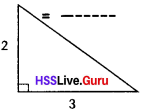Hypotenuse = $$\sqrt{13}; \sqrt{2^{2} + 5^{2}}=\sqrt{4 + 9}$$
Perimeter = 2 + 3 +$$\sqrt{13} = 5 + \sqrt{13}$$

Question 2.
Find the perimeter of the rectangle with area 10 sq. centimetres.
Let one side of the reactable be ‘a’
Area = a²
a² =10 sq. cm a = $$\sqrt{10}$$
Perimeter = 4 × a = 4 × $$\sqrt{10}$$ cm

Question 3.$$\sqrt{x} \times \sqrt{y} = \sqrt{x \times y} = \sqrt{x y}$$
$$\sqrt{2} \times \sqrt{7} = \sqrt{2 \times 7} = \sqrt{14}$$
$$\sqrt{8} = \sqrt{4} \times \sqrt{2} =\sqrt{4} \times \sqrt{2} = 2\sqrt{2}$$
$$\sqrt{18} = \sqrt{9} \times \sqrt{2} =\sqrt{9} \times \sqrt{2} = 3\sqrt{2}$$
$$\sqrt{8} + \sqrt{18} = 2\sqrt{2} + 3\sqrt{2} = 5\sqrt{2}$$

Question 4.
$$\frac{\sqrt{x}}{\sqrt {y}} = \frac{\sqrt{…..}}{\sqrt {…..}}$$
$$\frac{\sqrt{x}}{\sqrt {y}} = \frac{\sqrt{x}}{\sqrt {y}}$$

Question 5.
$$\frac{1}{\sqrt{2}} = \frac{1}{\sqrt{2}} \times \frac{\sqrt{2}}{\sqrt{2}} = \frac{\sqrt{2}}{…….}$$
$$\frac{\sqrt{1}}{\sqrt{2}} = \frac{\sqrt{1}}{\sqrt{2}} \times \frac{\sqrt{2}}{\sqrt{2}} = \frac{\sqrt{2}}{2}$$

Question 6.
Find the value of $$\sqrt 50$$ correct to 2 decimals.
$$\sqrt{50} = \sqrt{25 \times ……} = \sqrt{25} \times \sqrt{……} = 5\sqrt{2}$$
= 5 × 1.414 = ……
$$\sqrt{50} = \sqrt{25 \times 2} = \sqrt{25} \times \sqrt{2} = 5\sqrt{2}$$
= 5 × 1.414 = 7.070 = 7.07

Question 7.
Find the perimeter of the triangle correct to two, decimal places.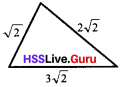Perimeter = $$\sqrt{2} + 2\sqrt{2} + 3\sqrt{2} = 6\sqrt{2}$$
= 6 × 1.414 = 8.484 = 8.48 cm

Question 8.
If x = $$\frac{1}{\sqrt{2}}$$, find (x + $$\frac{1}{x})^{2}$$
x = $$\frac{1}{\sqrt{2}}$$
$$\frac{1}{x} = \sqrt{2} ; \left(x + \frac{1}{x}\right)^{2} = \left(\sqrt{2} + \frac{1}{\sqrt{2}}\right)^{2}$$
2 + $$\frac{1}{2}$$ + 2 = $$4\frac{1}{2}$$ = 4.5

Question 9.
What is the total length of the line joining two lines of lengths $$\sqrt 2$$cm, $$\sqrt 3$$. Find the length correct to 3 decimal places.
Length of the line = $$\sqrt 2$$cm + $$\sqrt 3$$
= 1.414+ 1.732 = 3.146 cm

Question 10.
Which is greater $$\sqrt 3$$ + $$\sqrt 2$$, $$\sqrt 5$$cm?
$$\sqrt 3$$ + $$\sqrt 2$$
$$\sqrt 3$$ + $$\sqrt 2$$ = 3.146
$$\sqrt 5$$ = 2.236;
$$\sqrt 3$$ + $$\sqrt 2$$ + $$\sqrt 3$$ + $$\sqrt 2$$ > $$\sqrt 5$$.

Question 11.
A line of length $$\sqrt 27$$cm is cut from a line of length $$\sqrt 12$$cm. Find the length of the remaining part of the line?
Remaining part = $$\sqrt 27$$ – $$\sqrt 12$$
= $$3\sqrt 3$$ – $$2\sqrt 3$$ = $$\sqrt 3$$ cm

Question 12.
Simplify the following.
(a) $$\sqrt 50$$ × $$\sqrt 2$$
(b) $$\sqrt 27$$ × $$\sqrt 3$$
(c) $$\sqrt 12$$ × $$\sqrt 36$$
(d) $$\sqrt 24$$ × $$\sqrt 6$$
(e) $$\sqrt 28$$ × $$\sqrt 7$$
(f) $$\sqrt 32$$ × $$\sqrt 2$$
(g) $$\sqrt 5$$ × $$\sqrt 10$$ × $$\sqrt 2$$
(h) $$\sqrt 8$$ × $$\sqrt 5$$ × $$\sqrt 10$$
(a) $$\sqrt 25 × 2$$ x $$\sqrt 2$$ = $$\sqrt 25$$ × $$\sqrt 2$$ × $$\sqrt 2$$ = 5 × 2 = 10
(b) $$\sqrt 9 × 3$$ × $$\sqrt 3$$ = 3 × 3 = 9
(C) $$\sqrt 4 × 3$$ × 6 = 2 × $$\sqrt 3$$ × 6 = 12$$\sqrt 3$$
(d) $$\sqrt 6 × 4$$ × $$\sqrt 6$$ = 2 × 6 = 12
(e) $$\sqrt{4 \times 7} \times \sqrt{7} = 2 \times 7 = 14$$
(f) $$\sqrt{16 \times 2} \times \sqrt{2} = 4 \times 2 = 8$$
(g) $$\sqrt{5} \times \sqrt{5} \times \sqrt{2} \times \sqrt{2} = 10$$
(h) $$\sqrt{4} \times \sqrt{2} \times \sqrt{5} \times \sqrt{2} \times \sqrt{5} = 2 \times 2 \times 5 = 20$$

Question 13.
Simplify the following.
(a) $$3\sqrt{2} + 5 \sqrt{2}$$
(b) $$7\sqrt{5} – 3 \sqrt{5}$$
(C) $$4\sqrt{7} + 5 \sqrt{7} – 3\sqrt{7}$$
(d) $$2\sqrt{3} + \sqrt{27}$$
(e) $$4\sqrt{3} – 3 \sqrt{12} + 3\sqrt{75}$$
(f) $$\sqrt{162} – \sqrt{72}$$
(g) $$4\sqrt{12 }- \sqrt{50} – 5\sqrt{48}$$
(h) $$\sqrt{8}0 + \sqrt{125}$$
(i) $$\frac{1}{\sqrt{5} – 1}$$
(j) $$\frac{3}{2 \sqrt{3} – \sqrt{2}}$$
(k) $$\frac{5}{2 \sqrt{7} – 3\sqrt{5}}$$
(a) $$8\sqrt{2}$$

(b) $$4\sqrt{5}$$

(C) $$6\sqrt{7}$$

(d) $$\sqrt{3} + 3 \sqrt{3} = 5\sqrt{3}$$

(e) $$4\sqrt{3} – 3\sqrt{4 \times 3} + 3\sqrt{25 \times 3}$$
= 4 $$\sqrt{3} – 6\sqrt{3} + 15 \sqrt{3}$$
= $$13\sqrt 13$$

(f) $$\sqrt{81 \times 2} – \sqrt{36 \times 2} = 9\sqrt{2} – 6\sqrt{2} = 3\sqrt{2}$$

(g) $$4\sqrt{4 \times 3} – \sqrt{25 \times 2} – 5\sqrt{12 \times 4}$$
$$= 8\sqrt{3} – 5\sqrt{2} -20 \sqrt{2}$$
$$= -12\sqrt{3} – 5\sqrt{2}$$

(h) $$\sqrt{16 \times 5}+\sqrt{25 \times 5} = 4\sqrt{5} + 5 \sqrt{5} = 9\sqrt{5}$$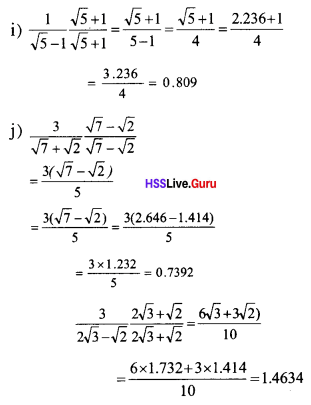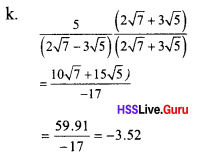Question 14.
If $$\sqrt{75} + \sqrt{363} + x\sqrt{3} = 0$$. Find the value of x.
$$x \sqrt{3} = 0 – \sqrt{75} – \sqrt{363}$$
$$= – 1 \times(\sqrt{75} + \sqrt{363})$$
$$x = \frac{-1(5 \sqrt{3} + 11 \sqrt{3})}{\sqrt{3}} = \frac{-1 \times 16 \sqrt{3}}{\sqrt{3}} = -16$$

Question 15.
Calculate $$\sqrt{3}(\sqrt{48} + \sqrt{32} – \sqrt{18})$$Question 16.
Write two fractions between $$\frac{1}{3}$$ and $$\frac {1}{4}$$
Fraction one = $$\frac{\frac{1}{3} + \frac{1}{4}}{2}=\frac{7}{24}$$
Another fraction =
$$\frac{\frac{1}{3} + \frac{7}{24}}{2} = \frac{8 + 7}{48} = \frac{15}{48}$$

Question 17.
In the figure given below, PQRS is a square of each side is 3 cm. Each sides of the square is divided into 3 equal parts. Joining these points to get an octagon, find its perimeter.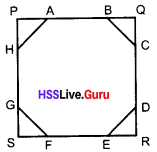ΔAPH is a right angled triangle.
AP = PH = 1 cm
∴ AH = $$\sqrt{1^{2}+1^{2}} = \sqrt{2}$$
AH = GF = ED = CB = $$\sqrt{2}$$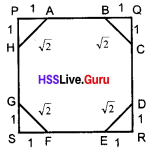Perimeter of the octagon
= AB + BC + CD + DE + EF + FG + GH + HA
$$=1 + \sqrt{2} + 1 + \sqrt{2} + 1 + \sqrt{2} + 1 + \sqrt{2} = 4 + 4 \sqrt{2}$$
= 4 + 4 × 1.41 = 4 + 5.64 = 9.64cm.

Question 18.
If three points A, B,C. Such that AB = $$\sqrt{50}$$cm, BC = $$\sqrt{98}$$cm, AC = $$\sqrt{288}$$cm. Check whether the points A,B,C lie on a straight line?
AB = $$\sqrt{50}$$ = $$5\sqrt{2}$$cm
BC = $$\sqrt{98}$$ = $$7\sqrt{2}$$cm
AC = $$\sqrt{288}$$ = $$12\sqrt{2}$$cm
$$5\sqrt{2}$$ + $$7\sqrt{2}$$ = $$12\sqrt{2}$$
AB + BC = AC
The three points lie on a straight line.

Question 19.
Find the value of $$\sqrt{12} – \frac{1}{\sqrt{3}}$$ correct to two decimal places.
$$\sqrt{12} – \frac{1}{\sqrt{3}} = \frac{2 \sqrt{3}}{1} – \frac{1}{\sqrt{3}} = \frac{6}{\sqrt{3}} – \frac{1}{\sqrt{3}} = \frac{5}{\sqrt{3}}$$
= $$\frac {5}{1.73}$$ = 2.89

Question 20.
Find the sum 745+7180+780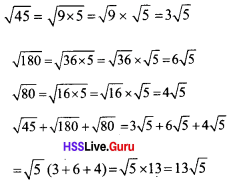A, B, C are three points such that AB = $$\sqrt {50}$$ cm, BC = $$\sqrt {98}$$ cm, AC =
$$\sqrt {288}$$ cm. Do they lie on a straightline?